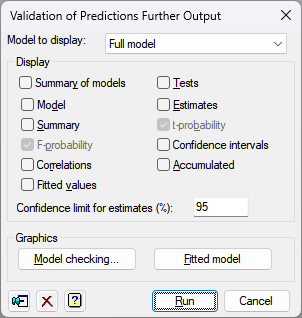1. Home
2. Validation of Predictions Further Output

# Validation of Predictions Further Output

Generates further output following a Validation of Predictions analysis.## Model to display

There are four models fitted in the analysis. Select which model for which the results are displayed.

 Full model The full model with the slope and constant/intercept estimated from the data Constant = 0 The model with the constant/intercept set to 0 and slope estimated from the data Slope = 1 The model with the slope set to 1 and constant/intercept estimated from the data Null model The null model with the slope set to 1 and constant/intercept set to 0

## Display

 Summary of models A summary of the model fits for the four models Tests Tests to assess whether the constant differs from zero, and the slope differs from one Model Model information Summary Summary analysis-of-variance F-probability F probabilities for variance ratios Correlations Correlations between the parameter estimates Fitted values Table containing the values of the response variate, the fitted values, standardized residuals and leverages Estimates Estimates of the parameters in the model t-probability t probabilities for the parameter estimates Confidence intervals Confidence intervals for the parameter estimates. The confidence limit can specified as a percentage using the Confidence limit for estimates (%) field. Accumulated Analysis of variance table containing a line for each change in the fitted model

The first two options give information on the four fitted models, and the rest on the selected model in the Model to display list.

## Model checking

You can generate diagnostic plots for the selected model.

## Fitted model

You can draw a graph of fitted model which shows the relationship of the observations and predictions for the selected model. For the Full and Constant = 0 models you will be prompted as to whether you want to include confidence limits in the plot. This confidence limits use the probability given in the Confidence limit for estimates (%) field.